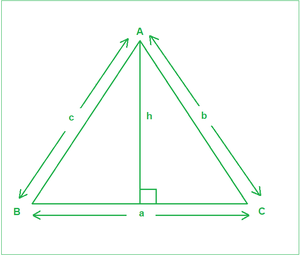Open in App
Not now

# Sine Rule with Derivation, Example and Implementation

• Last Updated : 13 Jul, 2021

Given angles(in degrees) A, C, and the side c, corresponding to the figure below, the task is to find the remaining two sides a and b.Examples:

Input: A = 45, C = 35, c = 23
Output:
28.35
39.49
Explanation:
a is 28.35 and b is 39.49

Input: A = 45, C = 45, c = 10
Output:
10
14.14

Approach: The idea is to use Sine rule. It states that the sides of any triangle are proportional to the sine of the angles opposite to them. a / Sin(A) = b / Sin(B) = c / Sin(C). The derivation is described below:

As is evident from the figure above:

A perpendicular of length h has been drawn on BC from A. From General trigonometric rules:

SinB=h/c——–(1)

SinC=h/b——–(2)

From the above two equations, we get:

c x SinB=b x SinC

Or b/SinB=c/SinC—–(3)

Similarly, if a perpendicular is drawn from B to AC, we can get:

a/SinA=c/SinC——-(4)

From Equations (3) and (4), we get:

a/SinA=b/SinB=c/SinC

Follow the steps below to solve the problem:

• Change the angles A and C from degrees to radians to be able to be used in the inbuilt functions.
• Calculate the angle B using the observation that sums of angles of a triangle sums up to 180 degrees.
• Use the Sine rule to calculate the sides a and b.

Below is the implementation of the above approach:

## C++14

 `// C++ program for the above approach` `#include ` `using` `namespace` `std;`   `// Function to calculate remaining two sides` `void` `findSides(``double` `A, ``double` `C, ``double` `c)` `{` `    ``// Calculate angle B` `    ``double` `B = 180 - (A + C);`   `    ``// Convert angles to their respective radians for` `    ``// using trigonometric functions` `    ``A = A * (3.14159 / 180);` `    ``C = C * (3.14159 / 180);` `    ``B = B * (3.14159 / 180);`   `    ``// Sine rule` `    ``double` `a = (c / ``sin``(C)) * ``sin``(A);` `    ``double` `b = (c / ``sin``(C)) * ``sin``(B);`   `    ``// Precision of 2 decimal spaces` `    ``cout << fixed << setprecision(2);`   `    ``// Print the answer` `    ``cout << a << endl;` `    ``cout << b << endl;` `}`   `// Driver Code` `int` `main()` `{` `    ``// Input` `    ``double` `A = 45.0;` `    ``double` `C = 35.0;` `    ``double` `c = 23;`   `    ``// Function Call` `    ``findSides(A, C, c);` `    ``return` `0;` `}`

## Java

 `// Java program for the above approach` `class` `GFG{`   `// Function to calculate remaining two sides` `static` `void` `findSides(``double` `A, ``double` `C,` `                      ``double` `c)` `{` `    `  `    ``// Calculate angle B` `    ``double` `B = ``180` `- (A + C);`   `    ``// Convert angles to their respective ` `    ``// radians for using trigonometric functions` `    ``A = A * (``3.14159` `/ ``180``);` `    ``C = C * (``3.14159` `/ ``180``);` `    ``B = B * (``3.14159` `/ ``180``);`   `    ``// Sine rule` `    ``double` `a = (c / Math.sin(C)) * Math.sin(A);` `    ``double` `b = (c / Math.sin(C)) * Math.sin(B);`   `    ``// Print the answer` `    ``System.out.println(String.format(``"%.2f"``, a));` `    ``System.out.println(String.format(``"%.2f"``, b));` `}`   `// Driver code` `public` `static` `void` `main(String[] args)` `{` `    `  `    ``// Input` `    ``double` `A = ``45.0``;` `    ``double` `C = ``35.0``;` `    ``double` `c = ``23``;`   `    ``// Function Call` `    ``findSides(A, C, c);` `}` `}`   `// This code is contributed by abhinavjain194`

## Python3

 `# Python3 program for the above approach` `import` `math`   `# Function to calculate remaining two sides` `def` `findSides(A, C, c):` `    `  `    ``# Calculate angle B` `    ``B ``=` `180` `-` `(A ``+` `C)`   `    ``# Convert angles to their respective radians` `    ``# for using trigonometric functions` `    ``A ``=` `A ``*` `(``3.14159` `/` `180``)` `    ``C ``=` `C ``*` `(``3.14159` `/` `180``)` `    ``B ``=` `B ``*` `(``3.14159` `/` `180``)`   `    ``# Sine rule` `    ``a ``=` `(c ``/` `math.sin(C)) ``*` `math.sin(A)` `    ``b ``=` `(c ``/` `math.sin(C)) ``*` `math.sin(B)`   `    ``# Precision of 2 decimal spaces`   `    ``# Print the answer` `    ``print``(``"{0:.2f}"``.``format``(a))` `    ``print``(``"{0:.2f}"``.``format``(b))`   `# Driver Code`   `# Input` `A ``=` `45.0` `C ``=` `35.0` `c ``=` `23`   `# Function Call` `findSides(A, C, c)`   `# This code is contributed by target_2`

## C#

 `// C# program for the above approach` `using` `System;` `class` `GFG{`   `// Function to calculate remaining two sides` `static` `void` `findSides(``double` `A, ``double` `C,` `                      ``double` `c)` `{` `    `  `    ``// Calculate angle B` `    ``double` `B = 180 - (A + C);`   `    ``// Convert angles to their respective ` `    ``// radians for using trigonometric functions` `    ``A = A * (3.14159 / 180);` `    ``C = C * (3.14159 / 180);` `    ``B = B * (3.14159 / 180);`   `    ``// Sine rule` `    ``double` `a = (c / Math.Sin(C)) * Math.Sin(A);` `    ``double` `b = (c / Math.Sin(C)) * Math.Sin(B);`   `    ``// Print the answer` `    ``Console.WriteLine(``"{0:F2}"``,a);` `    ``Console.WriteLine(``"{0:F2}"``,b);` `}`   `// Driver code` `public` `static` `void` `Main(String[] args)` `{` `    `  `    ``// Input` `    ``double` `A = 45.0;` `    ``double` `C = 35.0;` `    ``double` `c = 23;`   `    ``// Function Call` `    ``findSides(A, C, c);` `}` `}`   `// This code is contributed by shivanisinghss2110`

## Javascript

 ``

Output

```28.35
39.49```

Time Complexity: O(1)
Auxiliary Space: O(1)

My Personal Notes arrow_drop_up
Related Articles